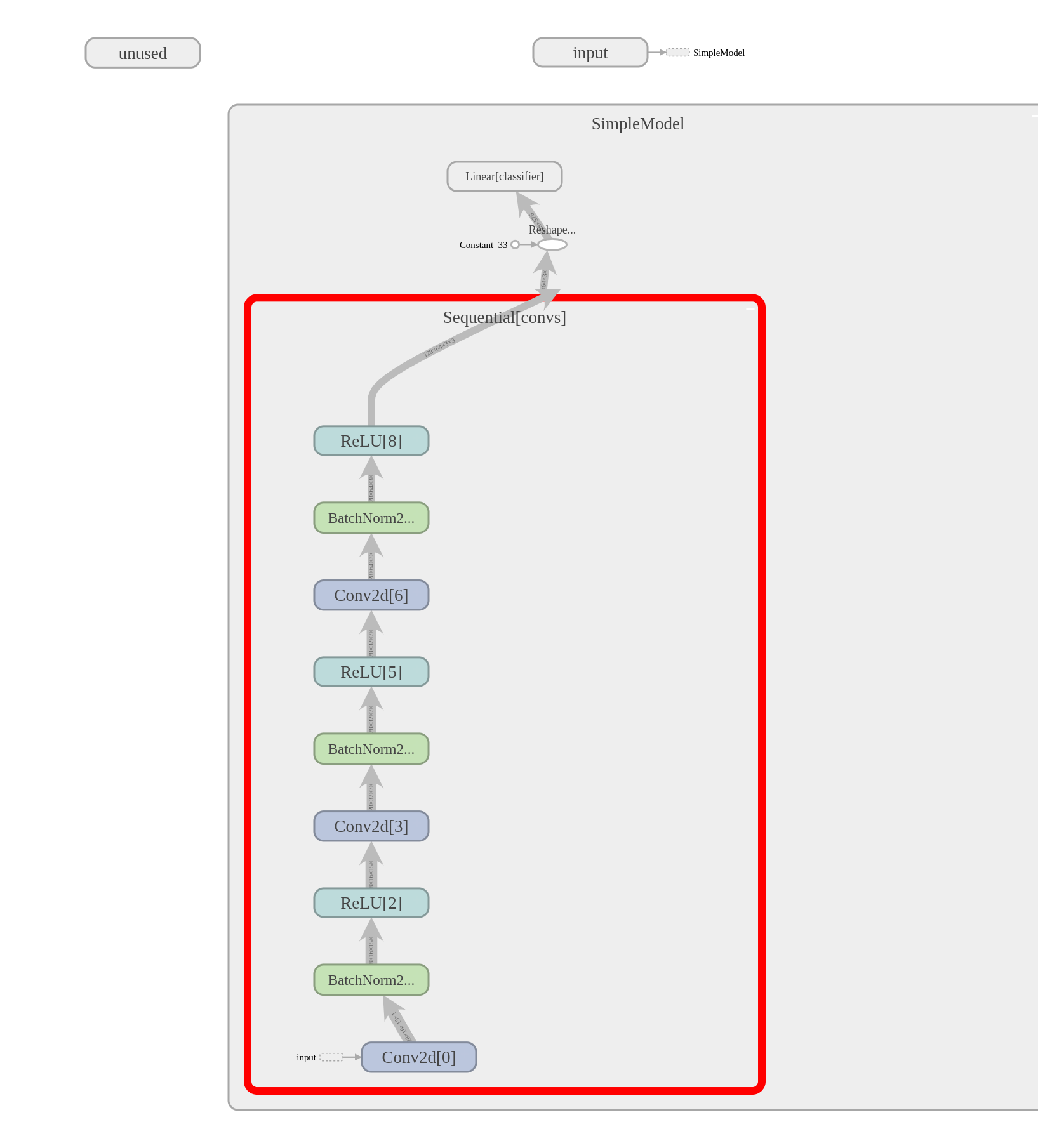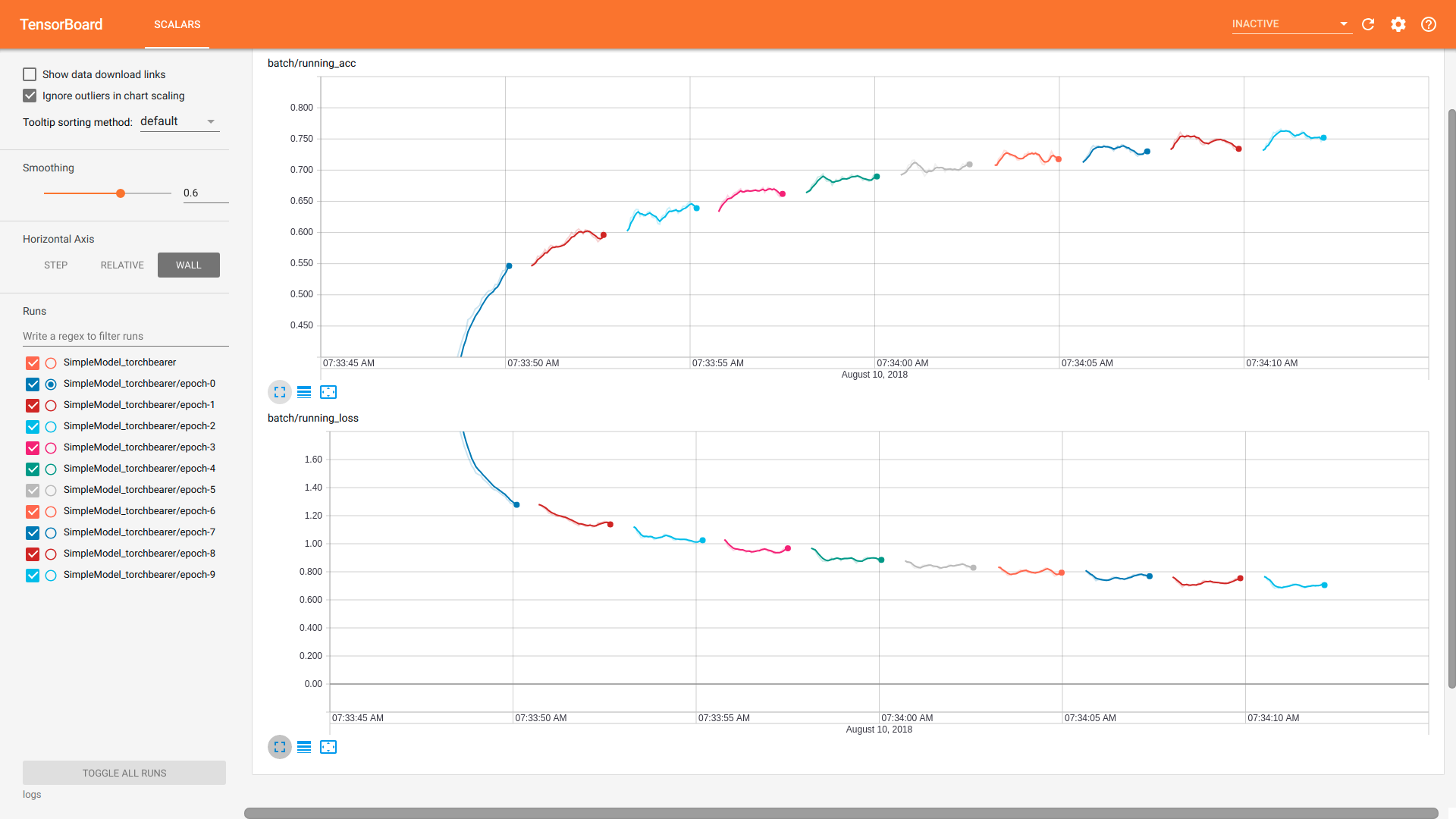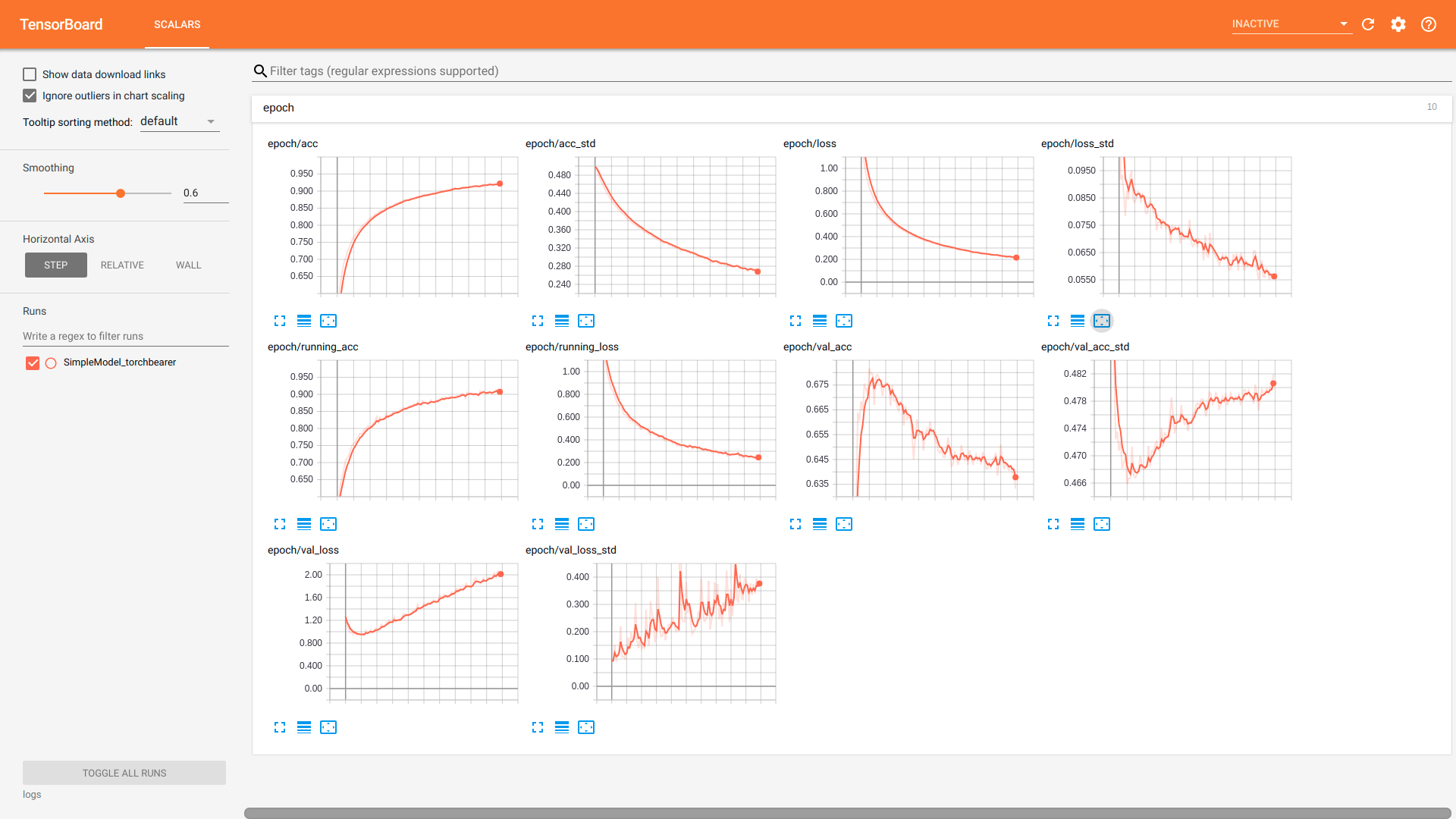# Using the Tensorboard Callback¶

In this note we will cover the use of the `TensorBoard callback`. This is one of three callbacks in torchbearer which use the TensorboardX library. The PyPi version of tensorboardX (1.4) is somewhat outdated at the time of writing so it may be worth installing from source if some of the examples don’t run correctly:

```pip install git+https://github.com/lanpa/tensorboardX
```

The `TensorBoard callback` is simply used to log metric values (and optionally a model graph) to tensorboard. Let’s have a look at some examples.

## Setup¶

We’ll begin with the data and simple model from our quickstart example.

```BATCH_SIZE = 128

normalize = transforms.Normalize(mean=[0.485, 0.456, 0.406],
std=[0.229, 0.224, 0.225])

transform=transforms.Compose([transforms.ToTensor(), normalize]))
splitter = DatasetValidationSplitter(len(dataset), 0.1)
trainset = splitter.get_train_dataset(dataset)
valset = splitter.get_val_dataset(dataset)

traingen = torch.utils.data.DataLoader(trainset, pin_memory=True, batch_size=BATCH_SIZE, shuffle=True, num_workers=10)
valgen = torch.utils.data.DataLoader(valset, pin_memory=True, batch_size=BATCH_SIZE, shuffle=True, num_workers=10)

transform=transforms.Compose([transforms.ToTensor(), normalize]))
testgen = torch.utils.data.DataLoader(testset, pin_memory=True, batch_size=BATCH_SIZE, shuffle=False, num_workers=10)
```
```class SimpleModel(nn.Module):
def __init__(self):
super(SimpleModel, self).__init__()
self.convs = nn.Sequential(
nn.Conv2d(3, 16, stride=2, kernel_size=3),
nn.BatchNorm2d(16),
nn.ReLU(),
nn.Conv2d(16, 32, stride=2, kernel_size=3),
nn.BatchNorm2d(32),
nn.ReLU(),
nn.Conv2d(32, 64, stride=2, kernel_size=3),
nn.BatchNorm2d(64),
nn.ReLU()
)

self.classifier = nn.Linear(576, 10)

def forward(self, x):
x = self.convs(x)
x = x.view(-1, 576)
return self.classifier(x)

model = SimpleModel()

loss = nn.CrossEntropyLoss()
```

The callback has three capabilities that we will demonstrate in this guide:

1. It can log a graph of the model
2. It can log the batch metrics
3. It can log the epoch metrics

## Logging the Model Graph¶

One of the advantages of PyTorch is that it doesn’t construct a model graph internally like other frameworks such as TensorFlow. This means that determining the model structure requires a forward pass through the model with some dummy data and parsing the subsequent graph built by autograd. Thankfully, TensorboardX can do this for us. The `TensorBoard callback` makes things a little easier by creating the dummy data for us and handling the interaction with TensorboardX. The size of the dummy data is chosen to match the size of the data in the dataset / data loader, this means that we need at least one batch of training data for the graph to be written. Let’s train for one epoch just to see a model graph:

```from torchbearer import Trial
from torchbearer.callbacks import TensorBoard

torchbearer_trial = Trial(model, optimizer, loss, metrics=['acc', 'loss'], callbacks=[TensorBoard(write_graph=True, write_batch_metrics=False, write_epoch_metrics=False)]).to('cuda')
torchbearer_trial.with_generators(train_generator=traingen, val_generator=valgen)
torchbearer_trial.run(epochs=1)
```

To see the result, navigate to the project directory and execute the command `tensorboard --logdir logs`, then open a web browser and navigate to localhost:6006. After a bit of clicking around you should be able to see and download something like the following:The dynamic graph construction does introduce some weirdness, however, this is about as good as model graphs typically get.

## Logging Batch Metrics¶

If we have some metrics that output every batch, we might want to log them to tensorboard. This is useful particularly if epochs are long and we want to watch them progress. For this we can set `write_batch_metrics=True` in the `TensorBoard callback` constructor. Setting this flag will cause the batch metrics to be written as graphs to tensorboard. We are also able to change the frequency of updates by choosing the `batch_step_size`. This is the number of batches to wait between updates and can help with reducing the size of the logs, 10 seems reasonable. We run this for 10 epochs with the following:

```torchbearer_trial = Trial(model, optimizer, loss, metrics=['acc', 'loss'], callbacks=[TensorBoard(write_graph=False, write_batch_metrics=True, batch_step_size=10, write_epoch_metrics=False)]).to('cuda')
torchbearer_trial.with_generators(train_generator=traingen, val_generator=valgen)
torchbearer_trial.run(epochs=10)
```

Runnng tensorboard again with `tensorboard --logdir logs`, navigating to localhost:6006 and selecting ‘WALL’ for the horizontal axis we can see the following:## Logging Epoch Metrics¶

Logging epoch metrics is perhaps the most typical use case of TensorBoard and the `TensorBoard callback`. Using the same model as before, but setting `write_epoch_metrics=True` we can log epoch metrics with the following:

```torchbearer_trial = Trial(model, optimizer, loss, metrics=['acc', 'loss'],  callbacks=[TensorBoard(write_graph=False, write_batch_metrics=False, write_epoch_metrics=True)]).to('cuda')
torchbearer_trial.with_generators(train_generator=traingen, val_generator=valgen)
torchbearer_trial.run(epochs=10)
```

Again, runnng tensorboard with `tensorboard --logdir logs` and navigating to localhost:6006 we see the following:Note that we also get the batch metrics here. In fact this is the terminal value of the batch metric, which means that by default it is an average over the last 50 batches. This can be useful when looking at over-fitting as it gives a more accurate depiction of the model performance on the training data (the other training metrics are an average over the whole epoch despite model performance changing throughout).

## Source Code¶

The source code for these examples is given below: